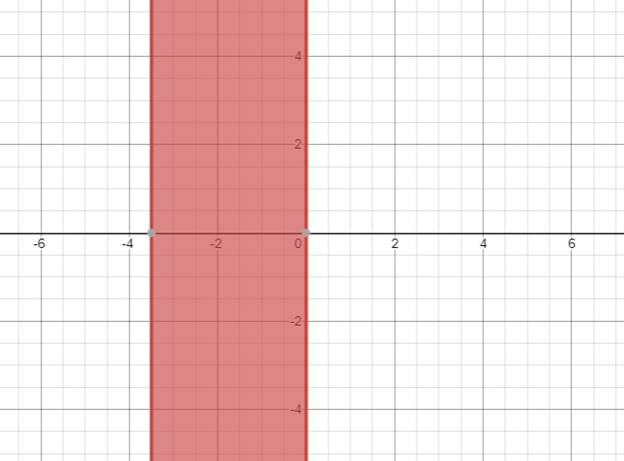# The solution of given nonlinear inequality equation and plot a graph for it.### Precalculus: Mathematics for Calcu...

6th Edition
Stewart + 5 others
Publisher: Cengage Learning
ISBN: 9780840068071### Precalculus: Mathematics for Calcu...

6th Edition
Stewart + 5 others
Publisher: Cengage Learning
ISBN: 9780840068071

#### Solutions

Chapter 1.7, Problem 37E
To determine

## The solution of given nonlinear inequality equation and plot a graph for it.

Expert Solution

3.5x0

### Explanation of Solution

Given:

Non-linear inequality equation

x(2x+7)0

Calculation,

Non-linear inequality equation,

x(2x+7)0(2x+7)02x7x72x3.5or,x0so,3.5x0

Now, graph of x(2x+7)0Conclusion:

Hence, the values of x lies between the -3.5 and 0.

### Have a homework question?

Subscribe to bartleby learn! Ask subject matter experts 30 homework questions each month. Plus, you’ll have access to millions of step-by-step textbook answers!